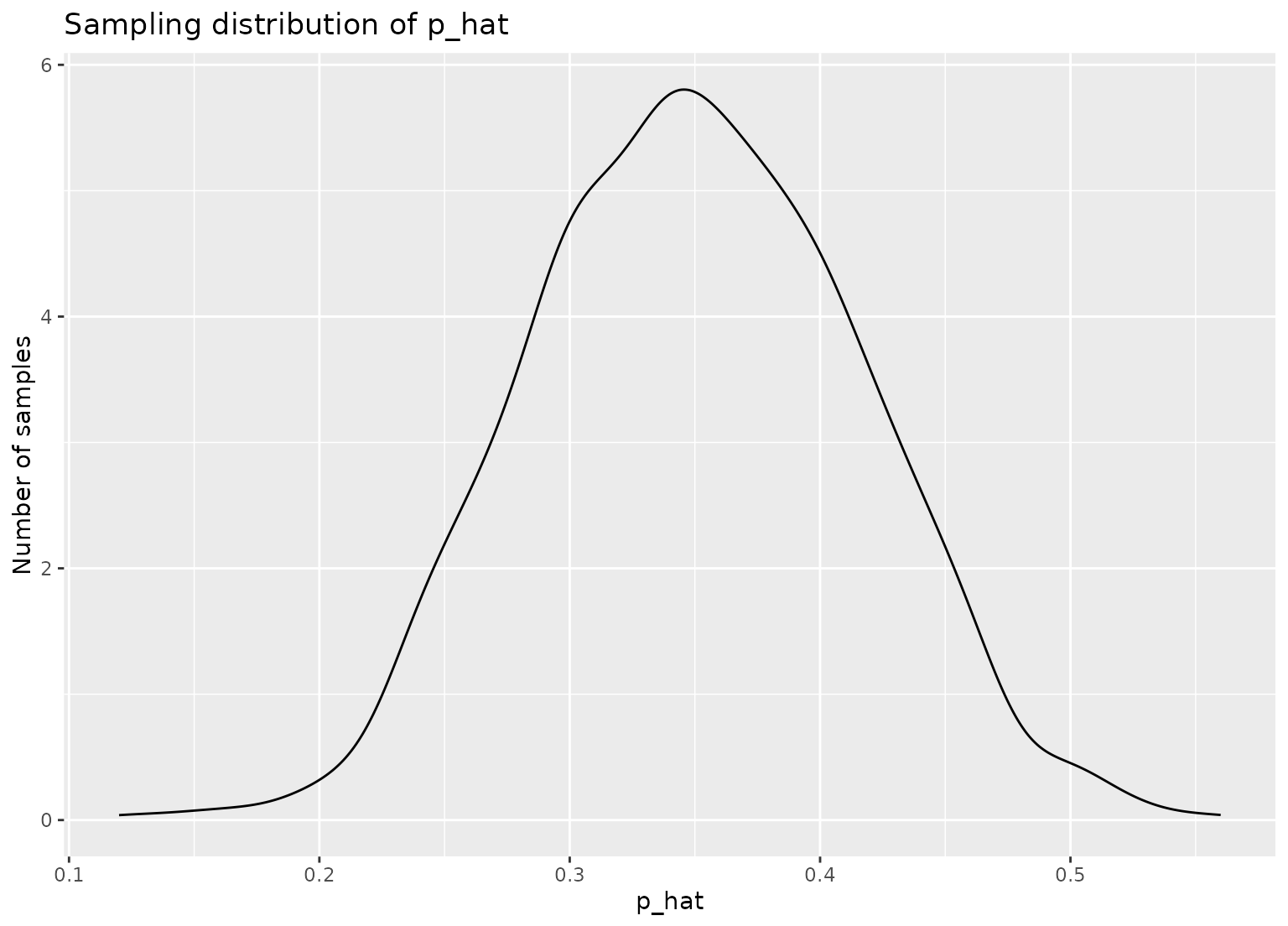These functions extend the functionality of dplyr::sample_n() and dplyr::slice_sample() by allowing for repeated sampling of data. This operation is especially helpful while creating sampling distributions—see the examples below!

rep_sample_n(tbl, size, replace = FALSE, reps = 1, prob = NULL)

rep_slice_sample(
.data,
n = NULL,
prop = NULL,
replace = FALSE,
weight_by = NULL,
reps = 1
)

## Arguments

tbl, .data Data frame of population from which to sample. size and n refer to the sample size of each sample. The size argument to rep_sample_n() is required, while in rep_slice_sample() sample size defaults to 1 if not specified. prop, an argument to rep_slice_sample(), refers to the proportion of rows to sample in each sample, and is rounded down in the case that prop * nrow(.data) is not an integer. When using rep_slice_sample(), please only supply one of n or prop. Should samples be taken with replacement? Number of samples to take. A vector of sampling weights for each of the rows in .data—must have length equal to nrow(.data).

## Value

A tibble of size reps * n rows corresponding to reps samples of size n from .data, grouped by replicate.

## Details

rep_sample_n() and rep_slice_sample() are designed to behave similar to their dplyr counterparts. As such, they have at least the following differences:

• In case replace = FALSE having size bigger than number of data rows in rep_sample_n() will give an error. In rep_slice_sample() having such n or prop > 1 will give warning and output sample size will be set to number of rows in data.

Note that the dplyr::sample_n() function has been superseded by dplyr::slice_sample().

## Examples

library(dplyr)
#>
#> Attaching package: ‘dplyr’
#> The following objects are masked from ‘package:stats’:
#>
#>     filter, lag
#> The following objects are masked from ‘package:base’:
#>
#>     intersect, setdiff, setequal, union
library(ggplot2)
library(tibble)

# take 1000 samples of size n = 50, without replacement
slices <- gss %>%
rep_slice_sample(n = 50, reps = 1000)

slices
#> # A tibble: 50,000 × 12
#> # Groups:   replicate [1,000]
#>    replicate  year   age sex   college partyid hompop hours income class finrela
#>        <int> <dbl> <dbl> <fct> <fct>   <fct>    <dbl> <dbl> <ord>  <fct> <fct>
#>  1         1  1998    27 fema… no deg… rep          3    40 $1500… work… average #> 2 1 2012 26 fema… no deg… ind 10 20$2500… work… below …
#>  3         1  1996    54 male  no deg… ind          4    30 $1500… work… below … #> 4 1 1993 39 fema… no deg… ind 6 37$2000… work… average
#>  5         1  2010    49 male  degree  ind          1    40 $2500… midd… average #> 6 1 1991 27 fema… degree rep 1 50$2500… midd… average
#>  7         1  1985    38 fema… no deg… dem          4    38 $8000… lowe… far be… #> 8 1 2000 37 male no deg… dem 3 50$2500… work… average
#>  9         1  2000    22 male  no deg… ind          2    15 $4000… midd… average #> 10 1 1998 45 male degree rep 1 50$2500… midd… average
#> # … with 49,990 more rows, and 1 more variable: weight <dbl>

# compute the proportion of respondents with a college
# degree in each replicate
p_hats <- slices %>%
group_by(replicate) %>%
summarize(prop_college = mean(college == "degree"))

# plot sampling distribution
ggplot(p_hats, aes(x = prop_college)) +
geom_density() +
labs(
x = "p_hat", y = "Number of samples",
title = "Sampling distribution of p_hat"
)# sampling with probability weights. Note probabilities are automatically
# renormalized to sum to 1
df <- tibble(
id = 1:5,
letter = factor(c("a", "b", "c", "d", "e"))
)

rep_slice_sample(df, n = 2, reps = 5, weight_by = c(.5, .4, .3, .2, .1))
#> # A tibble: 10 × 3
#> # Groups:   replicate 
#>    replicate    id letter
#>        <int> <int> <fct>
#>  1         1     1 a
#>  2         1     2 b
#>  3         2     1 a
#>  4         2     4 d
#>  5         3     1 a
#>  6         3     3 c
#>  7         4     2 b
#>  8         4     1 a
#>  9         5     1 a
#> 10         5     5 e